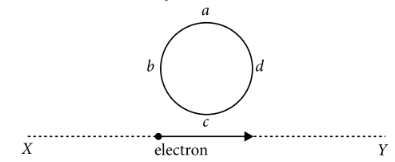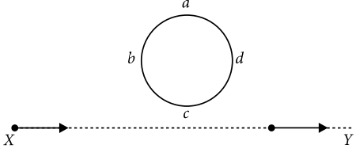Lenz's law and conservation of energy
Question

# An electron moves on a straight line path  X Y  as shown. The a b c d is a coil adjacent to the path of electron. What will be the direction of current, if any, induced in the coil?Moderate
Solution

##When the electron moves from X to Y, the flux linked with the coil a b c d (which is into the page) will first increase and then decrease as the electron passes by. So the induced current in the coil will be first anticlockwise and will reverse its direction (i.e. will become clockwise) as the electron goes past the coil.

Get Instant Solutions